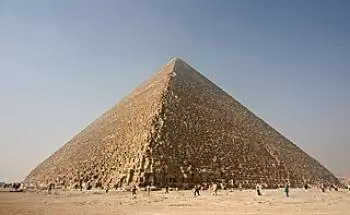# Square Pyramid: Number of Vertices, Edges, and Volume FormulaIn geometry, a square pyramid is an irregular polyhedron consisting of a square base and four triangular faces that meet at a common vertex point.

The pyramid's base and vertex are connected by the triangular faces that form the lateral faces of the pyramid.

As with any pyramid, the height of the square pyramid is the distance from the vertex to the base. The height is perpendicular to the plane of the base and forms a right angle with it.

This type of geometric body is found in many structures, everyday objects, architecture, and monumental structures such as the Great Pyramid of Giza.

## How Many Faces, Vertices, and Edges Does a Square Pyramid Have?

Below we list the essential characteristics of this type of pyramid:

• The base is a square, a polygon with four equal sides and four 90-degree right angles.

• It has four triangular faces, each of which has a common vertex at the tip of the pyramid, and its base is formed by one of the sides of the square at the bottom and one of the edges of the pyramid.

• It is an irregular polyhedron because at least one of its faces, the base, differs from the lateral faces formed by triangles.

• It has a common vertex at the top of the pyramid where the four triangular faces meet.

• It has eight edges, which are the lines that connect the vertices of the pyramid.

• It has five vertices, including the top vertex and the four vertices where the lateral edges of the pyramid meet the base.

## Volume of a Square Pyramid Formula

The volume of a square pyramid can be calculated using the formula:

V = (1/3) * l2 * h

where

• "l" is the length of one edge of the square that forms the base.

• "h" is the height measured from the base to the vertex.

## Historical References

The best-known square pyramid is the Great Pyramid of Giza, one of the Seven Wonders of the Ancient World. It was built during the reign of Pharaoh Cheops, around 2560 BC, and is the largest and oldest of the three main pyramids at Giza.

The construction of the Great Pyramid of Giza was an impressive achievement of ancient engineering. It is estimated that around 2.3 million stone blocks were used for its construction, each weighing about 2.5 tons.

Other important square pyramids include the Pyramid of the Moon in Teotihuacan, Mexico, and the Cholula Pyramid in Puebla, Mexico. Both pyramids were built by the pre-Hispanic cultures of Mesoamerica and were used as ceremonial centers.

The architecture of the square pyramid has also been used to construct modern buildings, such as the Palace of Culture and Science in Warsaw, Poland, and the Television Tower in Berlin, Germany.

Author:

Published: April 27, 2023
Last review: April 27, 2023